# 先说说Aster的TRN

As can be seen, the recogintion accuracy is farely even on words whose lengths are equal to or less than 11. Beyond this length, a drop in accuracy is observed.

# 接下来说说FA（Focus Attention）

2017.10的论文了，日期有点老，不过，我还是去啃了一下。

（…未完成）

# 由来##

• 把FCN语义分割方法引入进来，避免ctc那样的一维序列方式，哈哈，升维了（二维了）
• 引入了注意力机制，嫌语义分割还不够狠，再加上注意力，哈哈，想SOTA就要下猛剂啊
• 啥imprecise localization的模糊初始啥的，没搞明白，//TODO???

# TextScanner

TextScanner是旷视的姚神、华中科技大的白神坐阵的最新的一个识别力作，第一作者是旷视的万昭祎，第二作者是华中科技大的何明航。我就阅读论文中遇到的一些问题，向何明航进行了请教，得到了他很大帮助，这里特别感谢一下。

• G:Character Segmentation：W，H，Class（Class是字符集个数），这个用来分辨是哪个字符
• H:Order Segmentation：W，H，N（N是序列长度），这个是来分辨字符的从左往右的顺序
• Q:Localization Map：W，H，1，这个是用来告诉那些像素是字符像素

# 网络结构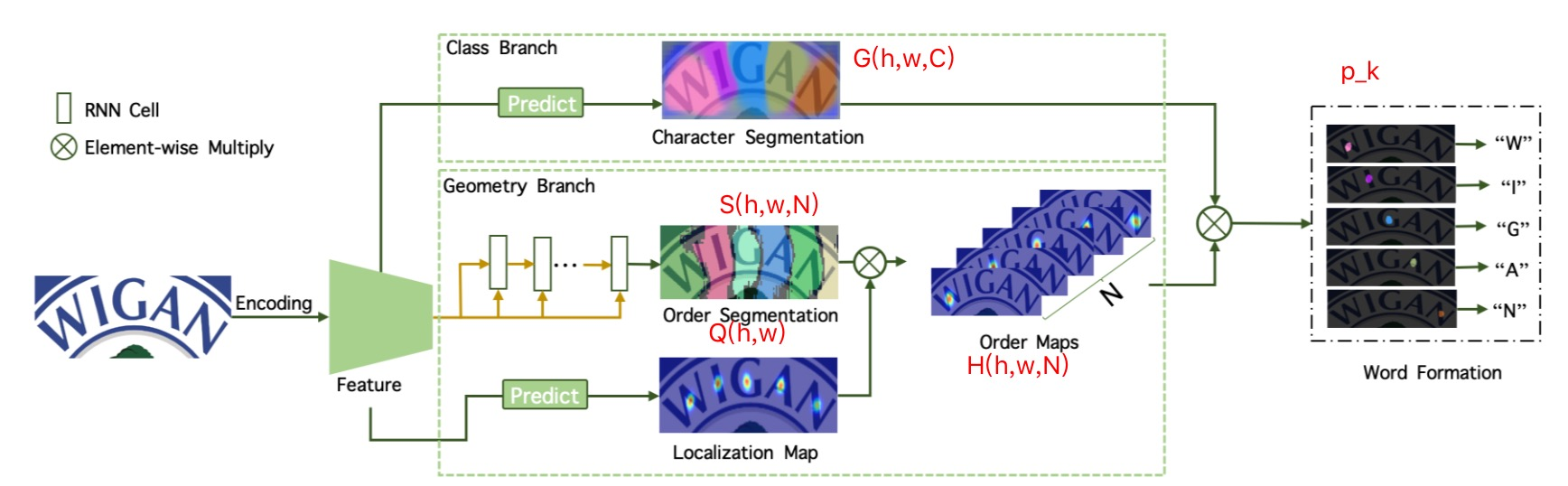• Character Segmentation：W，H，Class（Class是字符集个数）
• Order Segmentation：W，H，N（N是序列长度）
• Localization Map：W，H，1
• Order Maps：W，H，N

## Class Branch - Charachtor Segmenation（$G(w,h,class)$）

Our model is built on top of the backbone from CA-FCN, in which the character attentions are removed and VGG blocks are replaced with a ResNet-50(He et al. 2016) base model.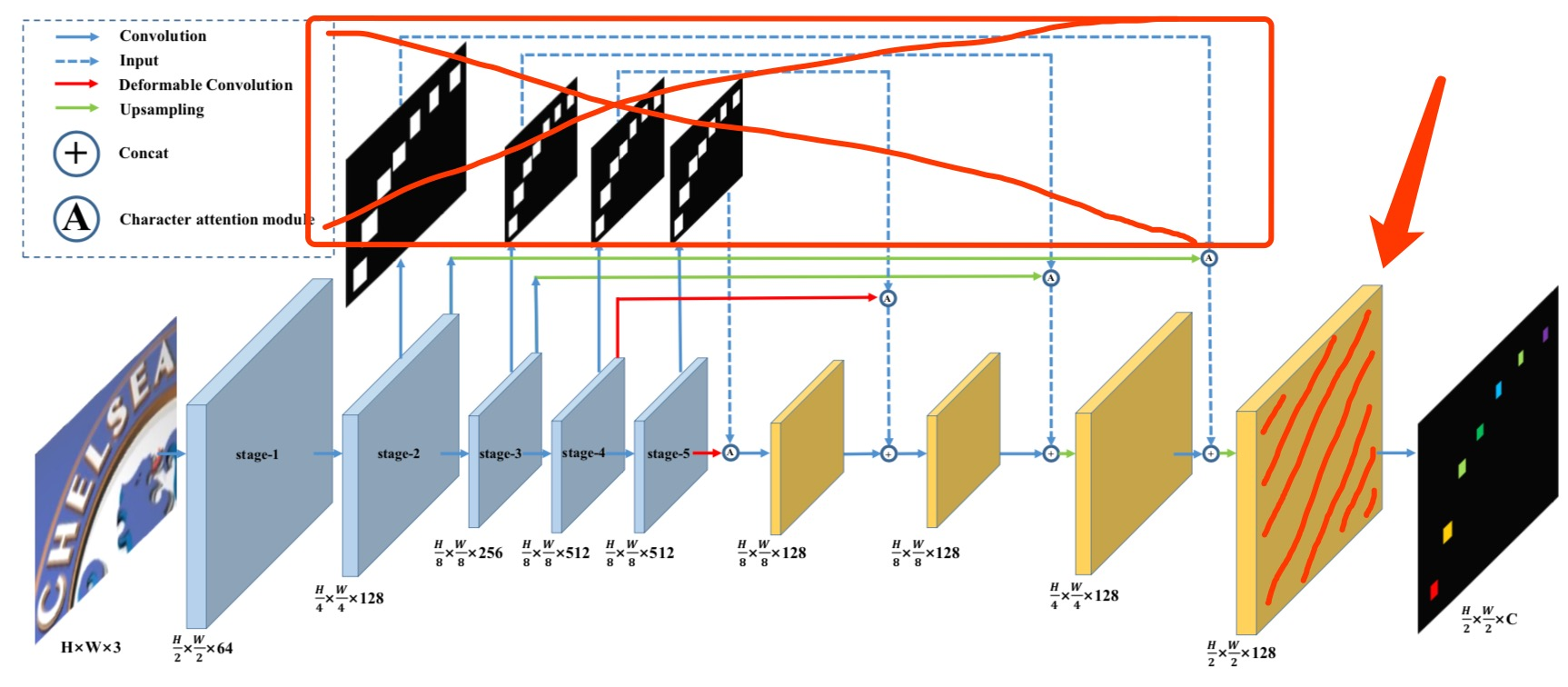During training and inference, the input im- ages are resized to 64 × 256.

The prediction module is composed of two stacked convolutional layers with kernel size 3×3 and 1×1.

## Geometry Branch - Localization Map（$G(w,h,1)$）

TODO:这个细节不知道推测的对不对，需要去问一下原论文作者？？？

## Geometry Branch - Order Segmentation（$S(w,h,N)$）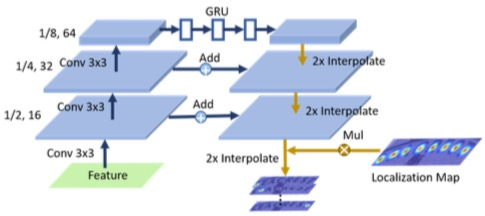Following the upsampling path, two convolutional layers are employed to generate the order segmenta- tion maps S。

## 最后大合体 Word Formation了

N就是字符的个数，论文里面是提到了N是设成30，也就是最多支持30个字符。

$p_k = \int_{(x,y)\in\Omega} G(x,y) * H_k(x,y)$

$G(x,y)$是一个$(h,w,C)$的东东，是个三维的，而$H_K(x,y)$是一个一维的，你是把要把这个一维的和三维的相乘后，求积分，就是求和啦，得到一个数，是一个概率值。

## 2020.6 补充

Word Formation确定的顺序就是字符的顺序。

$G维度是[H,W,C], H_k维度是[H,W,1]$，他们相乘后，得到的是维度是$[H,W,C]$，C就是字符集的个数，然后通过积分，也就是$\sum$，最终消掉了H和W，最终剩下的只有C。 所以，你最终得到的是一个维度为C的概率向量。

## 关于损失函数

$L=\lambda_l * L_l + \lambda_o * L_o + \lambda_m * L_m + L_s$

## 关于训练样本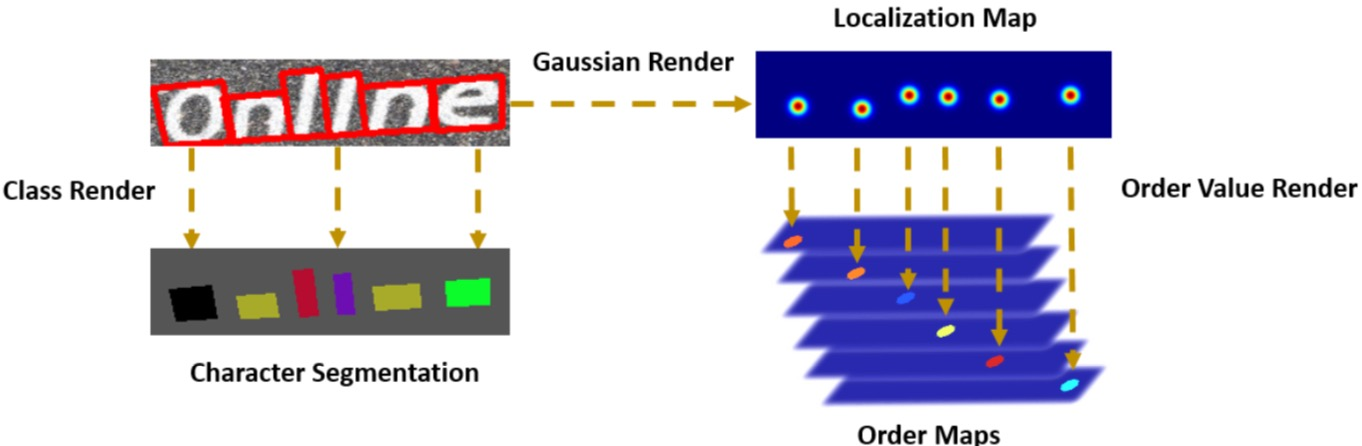• 先说说$L_s$需要的GT，即Character Sgementation（G）的GT：

Inside P’area,the class of the corresponding character is rendered as ground truth of the character segmentation.

其实就把标注的单字的标注框，shrink一下，然后得到的多边形里面，都填充成对应的字符，就得到了Character Segmentation的GT。当然，真正计算的损失的时候，还需要把这个字符转成one-hot向量。

实际得到的GT是一个（H,W,C+1），C是字库字符数，+1是要算上背景，且第三个C维度是一个one-hot编码的张量。

• 然后说说$L_o$所需要的GT，也就是Order Map（H），准确的是说，是每一个$H_k$的GT。

吐槽一下，这块论文里写的确实太晦涩了，真心看不懂，想了半天也没想清楚，情不得已，不得不向何明航同学请教，在他的帮助下，终于搞清楚了。

先看原文：

To generate the ground truth of order maps with character-level annotations, the center of Gaussian maps is firstly detected by computing the central points of characters bound- ing boxes. As Fig. 4 shown,2D Gaussian maps $\hat{Y}_k \in R^{h×w}$ with σ and expectation at central points are generated for each character. Then the order of characters is rendered for pixels inside $\hat{Y}_k$ area. Finally $\hat{Z}_k$ is normalized to [0, 1], to produce the ground truth $Z_k$ of $H_k$.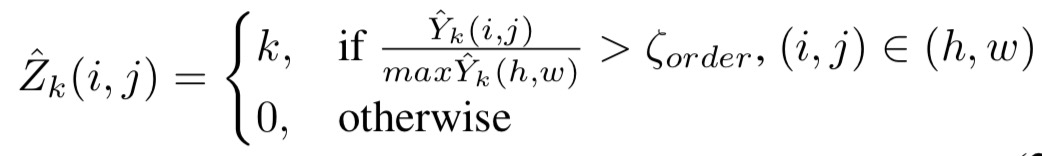有几处让人糊涂的地方：高斯分布，是用高斯方式采样一些样本点么？如果是一堆点的集合，那文中提到的$\hat{Y}_k$是点集么？那$\hat{Y}_k$的值又指的是什么？像素值么？$\max\hat{Y}_k$是像素值最大的值么？后面还提到要把$\hat{Z}_k$归一化，可是$\hat{Z}_k$的值都是k，怎么归一化？难道变成均匀分布的概率值？

带着这些问题，请教了何明航，终于得到了合理的解释：

最核心的是$\hat{Y}_k$的理解，它其实是一个高斯过滤图：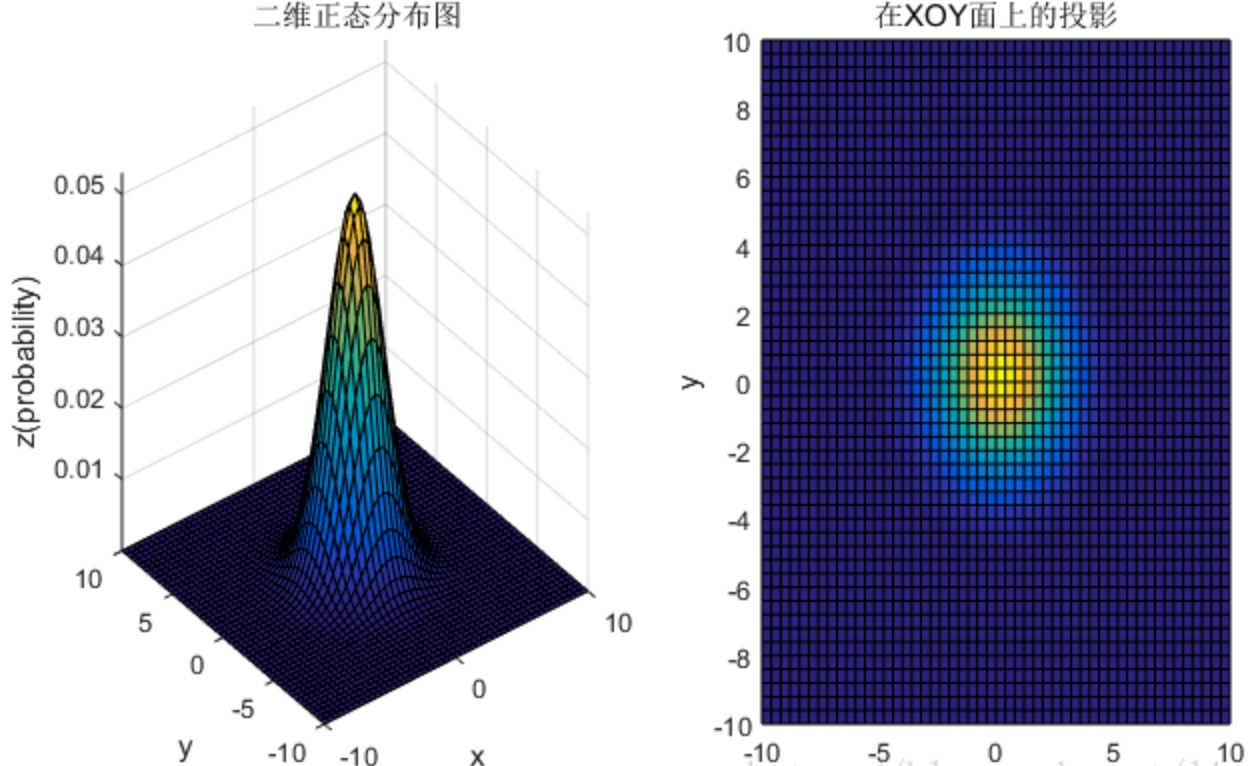他的值，其实就是概率密度函数的值，是一个概率值，凡是值比最大值小于0.5的点，值都被强制归0（就是上述公式里的处理）。 因为这些概率值都非常小，所以要做一个归一化（上面提到的）的动作，把值变到[0~1]之间，这个归一化动作也是有意为之的，因为，在Order Maps中的某个$H_k$，对应的区域就应该是文字区域，且这个区域表达的是“是前景文字的概率”，所以，归一化正好是为了满足这点。

这里我们需要思考一下，为何要这样做？也就是这个GT的insight：

前面提过，每个$H_k$对应是第几个字符的中心位置，按理说，应该是一个像素的位置值作为GT。但是，这里的设计是，用一个均值在这个字符的中心位置（方差是超参），来表达这个中心位置，这样做，我觉得，可能是因为文字块是一个区域，用一个正态分布来表示这个区域，越靠近中心区域给的权重越高。在网络预测时候，尽量让这个中心点预测为前景（文字）的概率最大，然后越往周边，预测为前景（文字）的概率逐渐decay，这样个设计，来帮助“确定”字符的中心区域。“对！用一种正态分布的方式来表达字的中心位置！”

再回忆一下论文中提到过，$H_k=S_k*Q$，$S_k$是order segement图[H,W,K]在axis=2上的切片，也就是对应的概率值（按照类别K进行softmax归一化的），这个切片图$S_k$然后要和一个$Q$相乘，$Q$是一个[H,W,1]，每个点的值是[0~1]的概率值，是经过sigmod计算后的，这样的两个数相乘，得到了$H_k$，所以$H_k$的数都是特别小的数。现在，我们就是要做这个特别小的值的$H_k$的样本来了。

理论上，在每个Order Segmentation的$S_k$对应的图上，第k个字符的那些点的标签的GT，softmax值都应该是1；而Localization Map（$Q$）的GT现在被我们把表示成了“正态分布归一化”的图。现在这两者相乘，也就是去模拟论文里$H_k=S_k*Q$的计算的话，那其实就是每个字符的“正态分布归一化”就是了。

这里，我是觉得论文的“《Label Generation》”的式（2）中，将$\hat{Z}_k = k$，这个赋值就很无厘头了，而且，后面论文也说了，要讲这个$\hat{Z}_k$还要归一化，就更说明这个式子是有问题的，其实，在我理解，就应该是归一化后的值，也就是$\frac{\hat{Y}_k}{max\hat{Y}_k}$

• 有了每个Order Map的每个$H_k$的GT，就可以制作Localization Map（Q）的GT了

其实就是把各个$H_k$合成到一起，不过细节上还是有些不太一样。

实现上，是把$\hat{Y}_k$归一化后（注意噢！要归一化），合并到一起，而不是直接$Z_k$合并到了一起。还记得$\hat{Y}_k$和$\hat{Z}_k$的区别么？$\hat{Z}_k$是去掉了一些概率值比较小点而已。

## 预测后的点（是某个字的点，是第几个位置的点）

Character Segmentation网络预测出一个$\hat{G}$，这个预测是每个像素是啥字符。如果我的这个字符串里的每个字符都是唯一的话，

$\Psi_h^k={(i,j)|\hat{H}(i,j)=k,Q(i,j)>\epsilon}$

$\Psi_g^k={(i,j)|\hat{H}(i,j)=k,G(i,j)>\epsilon}$

$\epsilon$=0.2

$\Psi_h^k$，就是拿着某个位置的汉字，去找这个对应位置的$H_k$图，然后根据Q的阈值，找出来是文字的点。

$\Psi_g^k$，就是拿着某个位置的汉字，去G中去找那些是这个汉字的点，然后再依据Q的阈值，找出这些点。

## 用这些点，相互校验，算损失

$L_g^k=\frac{1}{| \Psi_h^k |} \sum\limits_{(i,j)\in \Psi_g^k} L_{CE}\Big\lgroup G(i,j),onehot(T(k))\Big\rgroup$

$L_h^k=\frac{1}{| \Psi_g^k |} \sum\limits_{(i,j)\in \Psi_h^k} L_{CE}\Big\lgroup G(i,j),onehot(T(k))\Big\rgroup$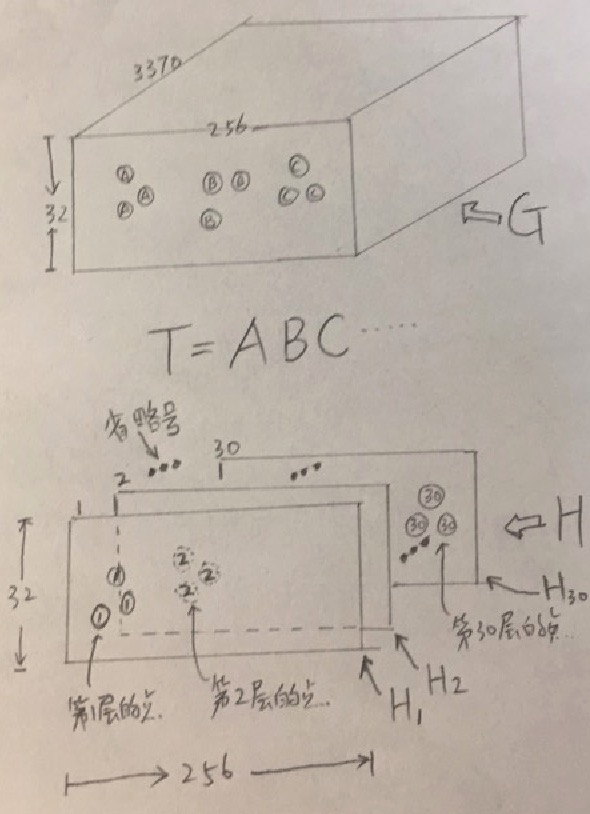T是字符序列。

$L_g^k=\frac{1}{| \Psi_h^k|} \sum\limits_{(i,j)\in \Psi_g^k} L_{CE}\Big\lgroup G(i,j),onehot(T(k))\Big\rgroup$

$L_h^k=\frac{1}{| \Psi_g^k|} \sum\limits_{(i,j)\in \Psi_h^k} L_{CE}\Big\lgroup G(i,j),onehot(T(k))\Big\rgroup$

## 提高置信度

$n_g^k= \left\{ \begin{array}{l} 1 , \quad if \quad \Psi_g^k \ne \emptyset, \\ 0 , \quad otherwise \end{array} \right.$ $\Phi_g = \frac{\sum_{k=1}^{\|T\|} n_g^k}{\|T\|}$

$n_h^k= \left\{ \begin{array}{l} 1 , \quad if \quad \Psi_h^k \ne \emptyset, \\ 0 , \quad otherwise \end{array} \right.$ $\Phi_h = \frac{\sum_{k=1}^{\|T\|} n_h^k}{\|T\|}$

## 最后，终于可以计算$L_m$了

$L_g=\frac{(\Phi_h)^\gamma}{|T|} \sum\limits_{k=1}^{|T|} L_g^k$

$\gamma=2$，$\gamma$是次方，不是简单的相乘啊，别看错了。

$L_h=\frac{(\Phi_g)^\gamma}{|T|} \sum\limits_{k=1}^{|T|} L_h^k$

$L_m=L_h + \lambda * L_g$

$\lambda=0.2$